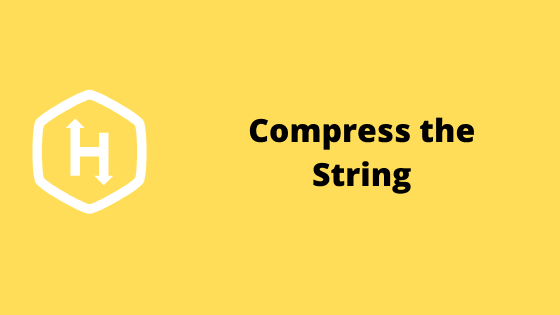Input Format
A single line of input consisting of the string .
Output Format
A single line of output consisting of the modified string.
Constraints

All the characters of  denote integers between  and .### Problem solution in python 2 programming language.

from __future__ import print_function
from itertools import *

for i,j in groupby(map(int,list(raw_input()))):
print(tuple([len(list(j)), i]) ,end = " ")

### Problem solution in python 3 programming language.

from itertools import *

for i,j in groupby(map(int,list(input()))):
print(tuple([len(list(j)), i]) ,end = " ")

#### Second solution

from itertools import groupby
for key, group in groupby(input()):
print('({}, {})'.format(len(list(group)), key), end=" ")